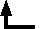## Dmitrii Mendeleev (1834-1907)

### On the Relationship of the Properties of the Elements to their Atomic Weights

D. Mendelejeff, Zeitschrift für Chemie 12, 405-406 (1869) translation from German by Carmen Giunta. Note: I have included hyperlinks from three of the blank places in this table to accounts of the discoveries of the corresponding elements at the ChemTeam site. I have left element symbols as they were in the German abstract. --CJG]

By ordering the elements according to increasing atomic weight in vertical rows so that the horizontal rows contain analogous elements, still ordered by increasing atomic weight, one obtains the following arrangement, from which a few general conclusions may be derived.

 Ti=50 Zr=90 ?=180 V=51 Nb=94 Ta=182 Cr=52 Mo=96 W=186 Mn=55 Rh=104,4 Pt=197,4 Fe=56 Ru=104,4 Ir=198 Ni=Co=59 Pd=106,6 Os=199 H=1 Cu=63,4 Ag=108 Hg=200 Be=9,4 Mg=24 Zn=65,2 Cd=112 B=11 Al=27,4 ?=68 Ur=116 Au=197? C=12 Si=28 ?=70 Sn=118 N=14 P=31 As=75 Sb=122 Bi=210? O=16 S=32 Se=79,4 Te=128? F=19 Cl=35,5 Br=80 J=127 Li=7 Na=23 K=39 Rb=85,4 Cs=133 Tl=204 Ca=40 Sr=87,6 Ba=137 Pb=207 ?=45 Ce=92 ?Er=56 La=94 ?Yt=60 Di=95 ?In=75,6 Th=118?
1. The elements, if arranged according to their atomic weights, exhibit a periodicity of properties.

2. Chemically analogous elements have either similar atomic weights (Pt, Ir, Os), or weights which increase by equal increments (K, Rb, Cs).

3. The arrangement according to atomic weight corresponds to the valence of the element and to a certain extent the difference in chemical behavior, for example Li, Be, B, C, N, O, F.

4. The elements distributed most widely in nature have small atomic weights, and all such elements are marked by the distinctness of their behavior. They are, therefore, the representative elements; and so the lightest element H is rightly chosen as the most representative.

5. The magnitude of the atomic weight determines the properties of the element. Therefore, in the study of compounds, not only the quantities and properties of the elements and their reciprocal behavior is to be taken into consideration, but also the atomic weight of the elements. Thus the compounds of S and Tl [sic--Te was intended], Cl and J, display not only many analogies, but also striking differences.

6. One can predict the discovery of many new elements, for example analogues of Si and Al with atomic weights of 65-75.

7. A few atomic weights will probably require correction; for example Te cannot have the atomic weight 128, but rather 123-126.

8. From the above table, some new analogies between elements are revealed. Thus Bo (?) [sic--apparently Ur was intended] appears as an analogue of Bo and Al, as is well known to have been long established experimentally.

(Russian Chemical Society 1, 60)Back to the list of selected historical papers.Back to the top of Classic Chemistry.